Next: NEW Up: usermanual Previous: Hamming filter   Contents

# INTERFERO

In this chapter the processing of step INTERFERO is described. In this step the following is computed.

The (complex) interferogram is computed, with or without subtraction of the reference phase. The reference phase is subtracted if there is a 2d-polynomial in the products result file (result of step FLATEARTH). It is not subtracted if this is not in the result file or if the number of coefficients is set to 0.

The complex interferogram minus reference phase is defined as: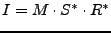(W1)

Where: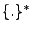denotes the complex conjugated;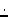denotes a pointwise multiplication;
I is the complex interferogram;
M is the complex master image;
S is the complex (resampled) slave image;
R is the complex (amplitude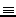1) reference phase.

The phase image (of the complex interferogram minus reference phase) is defined as: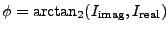(W2)

Where: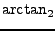is the four quadrant arc tangent;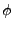is the phase image;
I is the complex interferogram;

This is identical to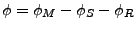(W3)

Multilooking can be performed to reduce noise. Usually a ratio of (line:pixel) = 5:1 between the factors is chosen to obtain more or less square pixels (20x20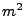for factors 5 and 1). (The resolution decreases of course if multilooking is applied.)

SubsectionsNext: NEW Up: usermanual Previous: Hamming filter   Contents
Leijen 2009-04-14